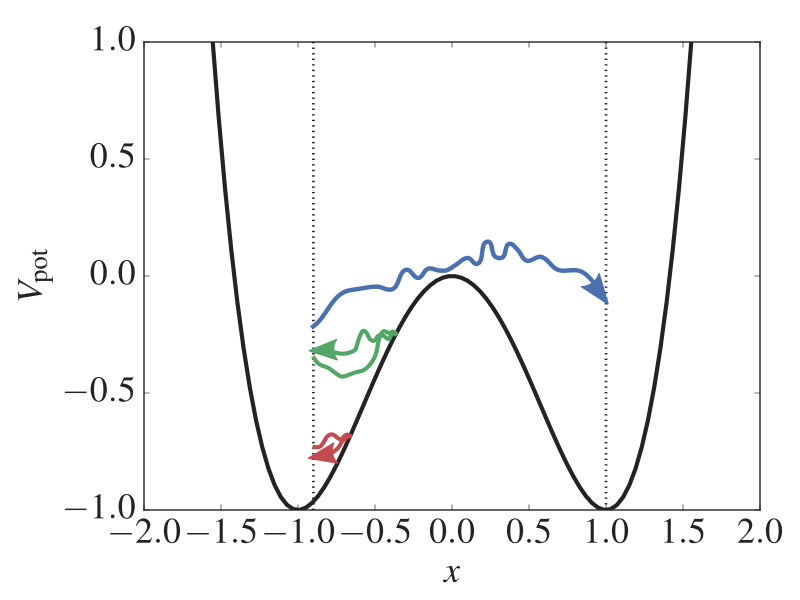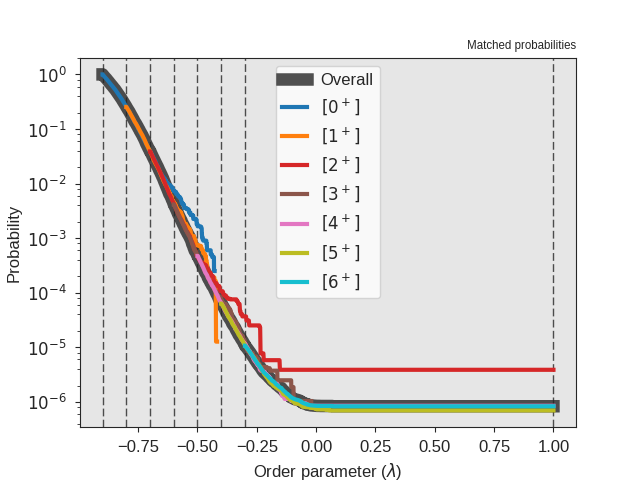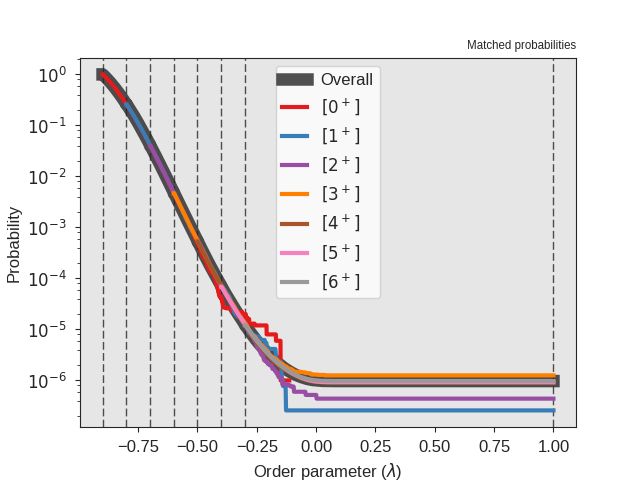# TIS in a 1D potential¶

In this example, you will explore a rare event with the Transition Interface Sampling (TIS) algorithm.

We will consider a simple 1D potential where a particle is moving. The potential is given bywhereis the position. By plotting this potential, we see that we have two states (atand) separated by a barrier (at):Fig. 18 The potential energy as a function of the position. We have two stable states (at x = -1 and x = 1) separated by a barrier (at x = 0). In addition, three paths are shown. One is reactive while the two others are not able to escape the state at x = -1. Using the TIS method, we can generate such paths which gives information about the reaction rate and the mechanism. The vertical dotted lines show two TIS interfaces.

Using the TIS algorithm, we will compute the rate constant for the transition between the two states. This particular problem has been considered before by van Erp   and we will here try to reproduce these results.

In order to obtain a rate constant, we will have to obtain both the crossing probability and an initial flux. To obtain to the crossing probability, we need to carry out TIS simulations for each path ensemble we define and in order to obtain the initial flux, we will have to carry out a MD simulation. Below we describe the two kinds of input files we need to create.

## Creating and running the TIS simulations with PyRETIS¶

We will now create the input file for PyRETIS. We will do this section by section in order to explain the different keywords and settings. The full input file is given at the end of this section.

### Setting up the simulation task¶

The first thing we will define is the type of simulation we will run. This is done by creating a simulation section.

Here, we are going to do a tis simulation and we will do 20000 steps . Since we will be running a path sampling simulation, we will also need to specify the positions of the interfaces we will be using.

TIS 1D example
==============

Simulation
----------
task = tis-multiple
steps = 20000
interfaces = [-0.9, -0.8, -0.7, -0.6, -0.5, -0.4, -0.3,  1.0]


### Setting up the system¶

We will now set up the system we are going to consider. Here, we will actually define several PyRETIS input sections:

• The system section which defines the units, dimensions and temperature we are considering:

System
------
units = reduced
dimensions = 1
temperature = 0.07

• The box section, but since we are here just considering a single particle in a 1D potential, we will simply use a 1D box without periodic boundaries:

Box
---
periodic = [False]

• The particles section which add particles to the system and defines the initial state:

Particles
---------
position = {'file': 'initial.xyz'}
velocity = {'generate': 'maxwell',
'momentum': False,
'seed': 0}
mass = {'Ar': 1.0}
name = ['Ar']
type = 


In this case, we will read the initial configuration from a file initial.xyz and velocities are generated from a Maxwell distribution. Further, we specify the mass, particle type and we label the particle as Ar. Note that this does not mean that we are simulating Argon, it is just a label used in the output of trajectories.

• The force field and potential sections which setup up the 1D double well potential:

Forcefield
----------
description = 1D double well

Potential
---------
class = DoubleWell
a = 1.0
b = 2.0
c = 0.0


### Selecting the engine¶

Here, we will make use of a stochastic Langevin engine. We set it up by setting the time step, the friction parameter and whether we are in the high friction limit. The seed given is a seed for the random number generator used by the integrator.

Engine
------
class = Langevin
timestep = 0.002
gamma = 0.3
high_friction = False
seed = 0


### TIS specific settings¶

The TIS settings control how the TIS algorithm is carried out. Here we set that 50 % of the moves should be shooting moves (keyword freq) and we limit all paths to a maximum length of 20 000 steps. Further, we select aimless shooting and we tell PyRETIS to not set the momentum to zero and to not rescale the energy after drawing new random velocities. We also set allowmaxlength = False which means that for shooting, we determine (randomly) the length of new paths based on the length of the path we are shooting from. The given seed is a seed for the random number generator used by the TIS algorithm.

TIS
---
freq =  0.5
maxlength = 20000
aimless = True
allowmaxlength = False
zero_momentum = False
rescale_energy = False
sigma_v = -1
seed = 0


### Initial path settings¶

These settings determine how we find the initial path(s). Here, we ask PyRETIS to generate these using the kick method.

Initial-path
------------
method = kick


### Selecting the order parameter¶

For this system, we simply define the order parameter as the position of the single particle we are simulating.

Orderparameter
--------------
class = Position
dim = x
index = 0
periodic = False


### Modifying the output¶

To save some disk space, we will set up the simulation to only write output for trajectories at every 100th step.

Output
------
energy-file = 100
order-file = 100
trajectory-file = 100


TIS 1D example
==============

Simulation
----------
task = tis-multiple
steps = 20000
interfaces = [-0.9, -0.8, -0.7, -0.6, -0.5, -0.4, -0.3,  1.0]

System
------
units = reduced
dimensions = 1
temperature = 0.07

Box
---
periodic = [False]

Engine
------
class = Langevin
timestep = 0.002
gamma = 0.3
high_friction = False
seed = 0

TIS
---
freq =  0.5
maxlength = 20000
aimless = True
allowmaxlength = False
zero_momentum = False
rescale_energy = False
sigma_v = -1
seed = 0

Initial-path
------------
method = kick

Particles
---------
position = {'file': 'initial.xyz'}
velocity = {'generate': 'maxwell',
'momentum': False,
'seed': 0}
mass = {'Ar': 1.0}
name = ['Ar']
type = 

Forcefield
----------
description = 1D double well

Potential
---------
class = DoubleWell
a = 1.0
b = 2.0
c = 0.0

Orderparameter
--------------
class = Position
dim = x
index = 0
periodic = False

Output
------
energy-file = 100
order-file = 100
trajectory-file = 100


### Running the TIS simulation(s)¶

We will now run the TIS simulation. Create a new directory and place the input file (let’s call it tis.rst) here. Also, download the initial configuration initial.xyz and place it in the same folder. The TIS simulations are executed in two steps:

1. Input TIS files are created for each path ensemble. This is done by running:

pyretisrun -i tis.rst


which will create files named tis-001.rst, tis-002.rst and so on. Each one of these files defines a TIS simulation for a specific path ensemble.

2. Running the PyRETIS TIS simulations for each path ensemble. The individual TIS simulations can be executed by running:

pyretisrun -i tis-001.rst -p -f 001.log


and so on. All these TIS simulations are independent and can, in priciple, be executed in parallel.

The -p option will display a progress bar for your simulation and -f will rename the log file.

### Analysing the TIS results¶

When the all the TIS simulations are finished, we can analyse the results. PyRETIS will create a file, out.rst_000, which you can use for the analysis. This is a copy of the input tis.rst with some additional settings for the analysis. For a description of the analysis specific keywords, we refer to the analysis section.

The analysis itself is performed using:

pyretisanalyse -i out.rst_000


This will produce a new folder, report which contains the results from the analysis. If you have latex installed, you can generate a pdf using the file tis_report.tex within the report folder. An example result is shown below.Fig. 19 The crossing probability after running for 20 000 cycles. The numerical value is.

## Creating and running the initial flux simulation with PyRETIS¶

The initial flux simulation measure effective crossings with the first interface. In PyRETIS such a simulation is requested by selecting the md-flux task.

This can be done by setting the following simulation settings:

Simulation settings
-------------------
task = md-flux
steps = 1000000
interfaces = [-0.9]


The other sections will be similar to the sections already defined for the TIS simulation, however, we do not need to include the TIS section for the md-flux simulation. The full input file is given below.

MD flux simulation
==================

Simulation settings
-------------------
task = md-flux
steps = 1000000
interfaces = [-0.9]

System settings
---------------
units = lj
dimensions = 1
temperature = 0.07

Engine settings
---------------
class = Langevin
timestep = 0.002
gamma = 0.3
high_friction = False
seed = 0

Particles
---------
position = {'file': 'initial.xyz'}
velocity = {'generate': 'maxwell',
'momentum': False,
'seed': 0}
mass = {'Ar': 1.0}
name = ['Ar']
type = 

ForceField settings
-------------------
description = 1D double well potential

Potential
---------
class = DoubleWell
a = 1.0
b = 2.0
c = 0.0

Orderparameter
--------------
class = Position
dim = x
index = 0
periodic = False

Output
------
backup = overwrite
energy-file = 1000
order-file = 1000
cross-file = 1
trajectory-file = -1


### Running the md-flux simulation¶

We will now run the md-flux simulation. Create a new directory and place the input file (let’s call it flux.rst) here. Also, download the initial configuration initial.xyz and place it in the same folder. The md-flux simulation can now be executed using:

pyretisrun -i flux.rst -p


### Analysing the md-flux results¶

When the md-flux simulation has finished, we can analyse the results. PyRETIS will create a file, out.rst, which you can use for the analysis. This is a copy of the input flux.rst with some additional settings for the analysis. For a description of the analysis specific keywords, we refer to the analysis section.

The analysis itself is performed using:

pyretisanalyse -i out.rst


This will produce a new folder, report which contains the results from the analysis. If you have latex installed, you can generate a pdf using the file md_flux_report.tex within the report folder. An example result is shown below.Fig. 20 The running average for the initial flux.

## Improving the statistics¶

We can improve the statistics by running a longer simulation. Modify the number of steps, from 20000 to 1000000 and re-run the simulation and the analysis. Below we show an example for the crossing probability after performing the additional stepsFig. 21 The crossing probability after running for 1 000 000 steps. The numerical value is.